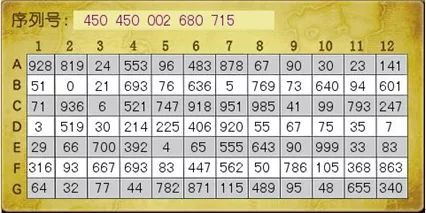# .NET Core 仿魔獸世界密保卡實現

《魔獸世界》的老玩家都知道，密保卡曾經被用於登入驗證，以保證賬號安全。今天我用.NET Core模擬了一把密保卡（也叫矩陣卡）的實現，分享給大家。（圖片來源於網路）.NET Core 實現Cell 類

Cell用於描述矩陣卡中的單元格。對於一個Cell，它擁有行標列標三個屬性。我分別用RowIndexColIndexValue來表示。為了方便顯示，我加入了ColumnName屬性，用於把列標顯示為英文字母（此處稍微和官方密保卡設計不一樣）。

public class Cell

{

public int RowIndex { get; }

public int ColIndex { get; }

public ColumnCode ColumnName => (ColumnCode)ColIndex;

public int Value { get; set; }

public Cell(int rowIndex, int colIndex, int val = 0)

{

RowIndex = rowIndex;

ColIndex = colIndex;

Value = val;

}

}

public enum ColumnCode

{

A = 0,

B = 1,

C = 2,

D = 3,

E = 4

}

ColumnCode 可以根據自己需要拓展，目前我只寫了5個值。

Card 類

Card用於描述一張密保卡。因此除了包含一堆Cell以外，還得有卡號（Id），以及行數、列數等資訊。起初的Card型別長這樣：

public class Card

{

public Guid Id { get; set; }

public int Rows { get; set; }

public int Cols { get; set; }

public List Cells { get; set; }

public Card(int rows = 5, int cols = 5)

{

Id = Guid.NewGuid();

Rows = rows;

Cols = cols;

Cells = new List();

}

}

[JsonIgnore]

public List Cells { get; set; }

public string CellData

{

get

{

var vals = Cells.Select(c => c.Value);

return string.Join(‘,’, vals);

}

}

private static int[,] GenerateRandomMatrix(int rows, int cols)

{

var r = new Random();

var arr = new int[rows, cols];

for (var row = 0; row < rows; row++)

{

for (var col = 0; col < cols; col++)

{

arr[row, col] = r.Next(0, 100);

}

}

return arr;

}

private void FillCellData(int[,] array)

{

for (var row = 0; row < Rows; row++)

{

for (var col = 0; col < Cols; col++)

{

var c = new Cell(row, col, array[row, col]);

}

}

}

private static void PrintCard(Card card)

{

Console.WriteLine(”  |\tA\tB\tC\tD\tE\t”);

Console.WriteLine(“———————————————-“);

var i = 0;

for (var k = 0; k < card.Rows; k++)

{

Console.Write(k + ” |\t”);

for (var l = 0; l < card.Cols; l++)

{

Console.Write(card.Cells[i].Value + “\t”);

i++;

}

Console.WriteLine();

}

}

{

var tempArrStr = strMatrix.Split(‘,’);

if (tempArrStr.Length != Rows * Cols)

{

throw new ArgumentException(

“The number of elements in the matrix does not match the current card cell numbers.”, nameof(strMatrix));

}

var arr = new int[Rows, Cols];

var index = 0;

for (var row = 0; row < Rows; row++)

{

for (var col = 0; col < Cols; col++)

{

arr[row, col] = int.Parse(tempArrStr[index]);

index++;

}

}

FillCellData(arr);

return this;

}

public IEnumerable PickRandomCells(int howMany)

{

var r = new Random();

for (var i = 0; i < howMany; i++)

{

var randomCol = r.Next(0, Cols);

var randomRow = r.Next(0, Rows);

var c = new Cell(randomRow, randomCol);

yield return c;

}

}

1. 在Cells中查詢具有同樣行列的單元格。

2. 對比這兩者的值是否相等，一旦遇到不相等直接傳回false，無需再驗證下一個單元格。

public bool Validate(IEnumerable cellsToValidate)

{

return (

from cell in cellsToValidate

let thisCell = Cells.Find(p => p.ColIndex == cell.ColIndex

&& p.RowIndex == cell.RowIndex)

select thisCell.Value == cell.Value)

.All(matches => matches);

}